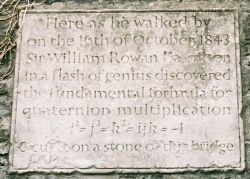#### You may also like### Roots and Coefficients

If xyz = 1 and x+y+z =1/x + 1/y + 1/z show that at least one of these numbers must be 1. Now for the complexity! When are the other numbers real and when are they complex?### Target Six

Show that x = 1 is a solution of the equation x^(3/2) - 8x^(-3/2) = 7 and find all other solutions.### 8 Methods for Three by One

This problem in geometry has been solved in no less than EIGHT ways by a pair of students. How would you solve it? How many of their solutions can you follow? How are they the same or different? Which do you like best?

# Two and Four Dimensional Numbers

##### Age 16 to 18Challenge LevelTo do this problem you only need to know how to add and multiply two by two matrices. The problem gives models for complex numbers and 4-dimensional numbers called quaternions. Although you don't need the information to do this problem you may like to read the NRICH article What are Complex Numbers? and the Plus article: Curious Quaternions .

(1) Let $C^*$ be the set of $2 \times2$ matrices of the form $$\left( \begin{array}{cc} x& -y\\ y& x\end{array} \right)$$ where $x$ and $y$ are real numbers and addition and multiplication are defined according to the usual rules for adding and multiplying matrices.

(a) Add and multiply the matrices $$\pmatrix {x & -y \cr y & x} {\rm \ and }\ \pmatrix {u & -v \cr v & u}.$$ (b) What are the identities and inverses for addition and multiplication?

(c) Consider also the subset $R^*$ for which $y=0$. Investigate the arithmetic of $R^*$ (addition, subtraction, multiplication, division, identities, inverses, distributive law) and compare it with the arithmetic of real numbers.

(d) Compare the arithemetic of $C^*$ with that of complex numbers.

(e) The matrix $\pmatrix {-1 & 0 \cr 0 & -1}$ is equivalent to the real number -1. What is the matrix equivalent to $i = \sqrt {-1}$ in the set of complex numbers?

(2) Complex numbers are two-dimensional numbers $x + iy$ where $x$ and $y$ are real numbers and $i = \sqrt {-1}$. Quaternions are four-dimensional numbers of the form $a+{\bf i}x+{\bf j}y+{\bf k}z$ where $a, x, y, z$ are real numbers and ${\bf i, j, k}$ are all different square roots of $-1$.

Can such a number system exist? To answer this, let's assume that in part (1) you have established that if real numbers and 2 by 2 matrices exist then so does the complex number $i = \sqrt {-1}$. The model for the system of quaternions is the set of linear combinations of 2 by 2 matrices: $$aI +{\bf i}x + {\bf j}y + {\bf k}z\ = a\pmatrix {1 & 0\cr 0 & 1}+ x\pmatrix {i & 0\cr 0 & -i}+y\pmatrix {0 & 1\cr -1 & 0}+ z\pmatrix {0 & i\cr i & 0}.$$ where $a, x, y, z$ are real numbers.

(a) Work out the matrix products: ${\bf i^2, \ j^2}$ and ${\bf k^2}$ showing that these matrices give models of three different square roots of -1.

(b) Work out the matrix products: ${\bf i j}$, ${\bf j i}$, ${\bf j k}$, ${\bf k j}$, ${\bf k i}$ and ${\bf i k}$ showing that the three matrices ${\bf i,\ j,\ k}$ model unit vectors along the three axes in 3-dimensional space ${\bf R^3}$ with matrix products isomorphic to vector products in ${\bf R^3}$.

You have now shown that this set of linear combinations of matrices models the quaternions.

(3) Investigate the sequence: ${\bf i},\$ ${\bf i j},\$ ${\bf i j}$ ${\bf k},\$ ${\bf i j}$ ${\bf k i},\$ ${\bf i j}$ ${\bf k i}$ ${\bf j,\ ...}$

... and the story continues in the Plus article: Ubiquitous Octonions